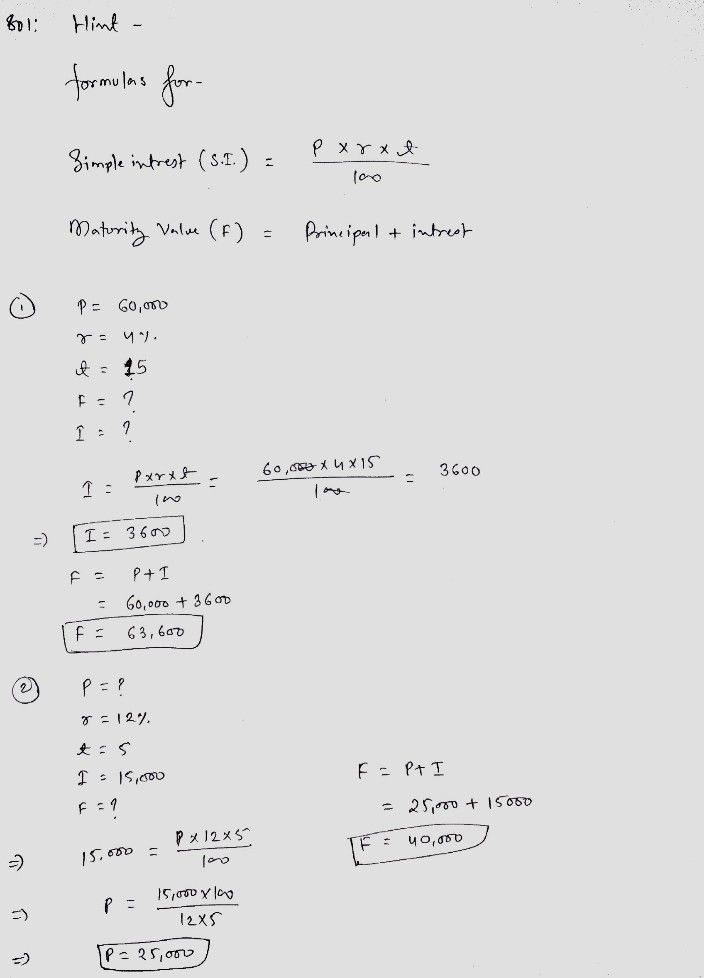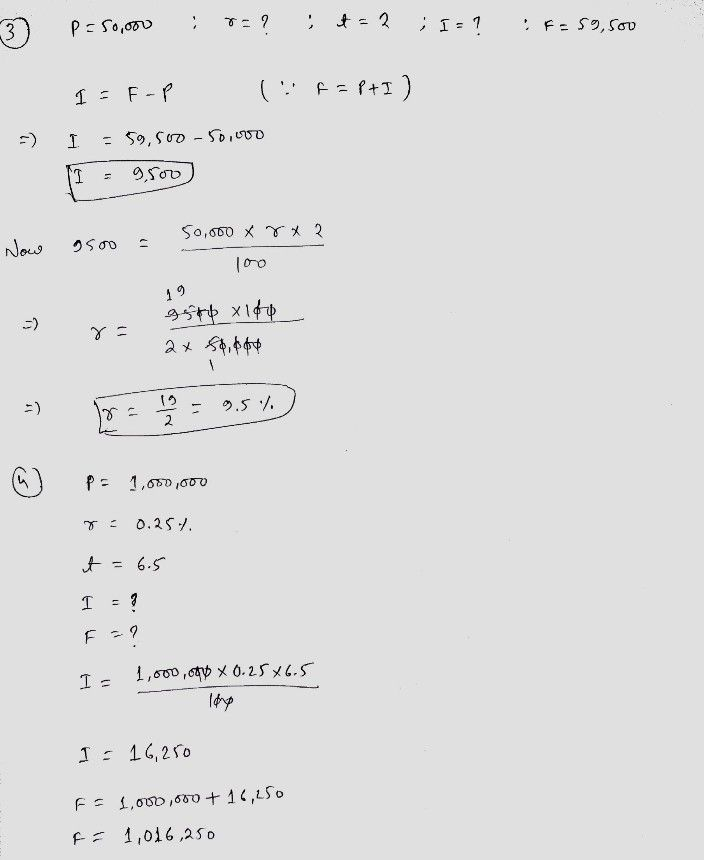Symbol
ProblemActivity 2 Complete the table by finding the unknown. Principal $\left(P\right)$ Rate (r) Time (t) | Interest (I) Maturity Value (F) $60,00$ 4% 15 $\left(1\right)$ (2) (3) $12%$ $15,000$ $\left(4\right)$ $50,00$ $\left(5\right)$ 2 $\left(6\right)$ $59,500$ $\left(7\right)$ $10.5%$ $\left(8\right)$ $157,500$ $457,500$ $1,000,0$ $0.25%$ 6.5 (9) $\left(10\right)$
10th-13th grade
Other
Search count: 132
Question content
answers only :) thank you
SolutionQanda teacher - PriyaHi, If you have any DOUBT regarding given solution, please feel free to DISCUSS.Student
no, it's perfect, thank you for helping me :)